# ISEE Upper Level Quantitative : Data Analysis

## Example Questions

### Example Question #41 : Data Analysis And Probability

Two standard decks of cards are altered as follows: ten cards from Deck 1 are switched with ten cards from Deck 2.

Which is the greater quantity?

(a) The probability that a card randomly drawn from Deck 1 is black

(b) The probability that a card randomly drawn from Deck 2 is red

It is impossible to tell from the information given

(b) is greater

(a) and (b) are equal

(a) is greater

(a) and (b) are equal

Explanation:

Since each deck will still have 52 cards, the number of red cards in Deck 1 will be equal to the number of black cards in Deck 2, and vice versa. Therefore, the two probabilities are the same.

### Example Question #25 : How To Find The Answer From A Table

Below is a table that gives the population of Washington City for five census years.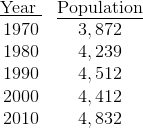Which is the greater quantity?

(a) The population increase in Washington City from 1970 to 1980

(b) The population increase in Washington City from 1980 to 1990

(a) is greater

It is impossible to tell from the information given

(b) is greater

(a) and (b) are equal

(a) is greater

Explanation:

(a) Between 1970 and 1980, the population increase was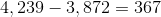(b) Between 1980 and 1990, the population increase was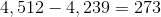(a) is greater

### Example Question #26 : How To Find The Answer From A Table

Below is a table that gives the population of Washington City for five census years.Which is the greater quantity?

(a) The mean increase in population per year from 1970 to 2010

(b) The mean increase in population per year from 1970 to 1980

It cannot be determined from the information given.

(a) and (b) are equal.

(a) is greater.

(b) is greater.

(b) is greater.

Explanation:

(a) The mean increase in population per year from 1970 to 2010 is the difference in the populations divided by 40: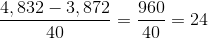(b) The mean increase in population per year from 1970 to 1980 is the difference in the populations divided by 10: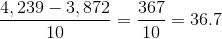(b) is greater.

### Example Question #23 : How To Find The Answer From A Table

Below is a table that gives the population of Washington City for five census years.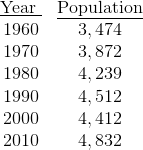Which is the greater quantity?

(a) The increase in the population of Washington City from 1980 to 1990

(b) The decline in the population of Washington City from 1990 to 2000

(b) is greater.

(a) and (b) are equal.

(a) is greater.

It is impossible to tell from the information given.

(a) is greater.

Explanation:

The population of Washington City was greater in 2000 than it was in 1980, so the decline over the second decade must have been less than the increase over the first.

### Example Question #28 : How To Find The Answer From A Table

John and James each have a standard deck of 52 cards. Each draws a card at random from his deck; John shows James a nine. Which is the greater quantity?

(a) The probability that James has a card higher in rank, aces being highest.

(b)(a) and (b) are equal.

(b) is greater.

(a) is greater.

It is impossible to tell from the information given.

(a) is greater.

Explanation:

To have a card higher in rank than John's nine, James must have a ten, a jack, a queen, a king, or an ace. This makes five ranks out of thirteen, each of which can be drawn with equal probability. James has a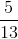probability of outranking John's card.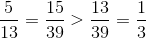, so (a) is greater.

### Example Question #41 : Data Analysis

Two fair eight-sided dice are altered so that each shows 1, 1, 1, 1, 2, 2, 3, 3 on its faces. The dice are tossed and the sum is noted.

Which is the greater quantity?

(a) The probability that a 2 will be tossed

(b) The probability that a 3 will be tossed

(a) and (b) are equal.

(a) is greater.

(b) is greater.

It is impossible to tell from the information given.

(a) and (b) are equal.

Explanation:

(a) For a 2 to be tossed, both dice must show a 1. There are four ways to roll a 1 on each die, so there are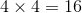ways to toss a 2.

(b) For a 3 to be tossed, one die must show a 1 and the other must show a 2. There are four ways to toss a 1 on the first die and two ways to toss a 2 on the second, so there are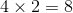ways to toss this; by symmetry, there are 8 ways to toss a 2 and a 1, since the dice are alike. This makes a total of 16 ways to toss a 3.

Since the dice are fair, the probabilities are equal.

### Example Question #42 : Data Analysis

Two fair dice are thrown. Which is the greater quantity?

(a) The probability that the sum wiil be a prime number

(b)(b) is greater.

It is impossible to tell from the information given.

(a) is greater.

(a) and (b) are equal.

(a) is greater.

Explanation:

The prime numbers that can be the sum of the dice are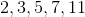.

The following rolls result in one of these outcomes: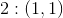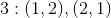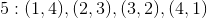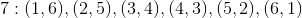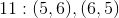15 rolls out of a possible 36 result in prime sums.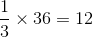Therefore (a) is greater.

### Example Question #43 : Data Analysis

Two fair dice are thrown. Which is the greater quantity?

(a) The probability that one die will show a number one more than the other

(b) The probability that one die will show a number two more than the other

(a) and (b) are equal.

It is impossible to tell from the information given.

(a) is greater.

(b) is greater.

(a) is greater.

Explanation:

(a) The following rolls result in one die being one higher than the other: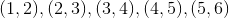, and the reverse of each of these.

Therefore there are ten possible rolls.

(b) The following rolls result in one die being two higher than the other: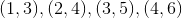, and the reverse of each of these.

Therefore there are eight possible rolls.

This makes (a) greater.

### Example Question #42 : Data Analysis And Probability

Two fair eight-sided dice from a role-playing game are marked with the numbers from 1 to 8. The two dice are tossed and their sum is noted.

Which is the greater quantity?

(a) The probability that the sum will be 6 or less

(b)It is impossible to tell from the information given.

(b) is greater.

(a) is greater.

(b) is greater.

(b) is greater.

Explanation:

There are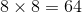possible rolls of the two dice. The ones that result in a sum of 6 or less are: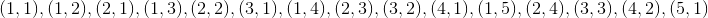This makes 15 rolls. Since one-fourth of 64 is 16, the probability of one of these rolls is less than.

### Example Question #45 : Data Analysis

The table below gives the population of Buchanan City in each census year from 1930 to 1980.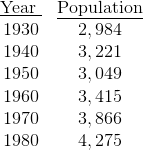It is known that the population increased from 1945 to 1950.

Which is the greater quantity?

(a) The population of Buchanan City in 1940

(a) The population of Buchanan City in 1945

(a) is greater.

(b) is greater.

It is impossible to tell from the information given.

(a) and (b) are equal.